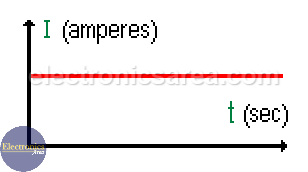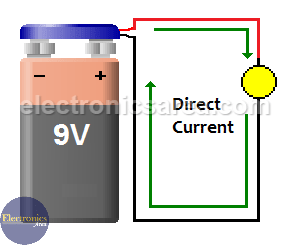# What is Direct Current? – DC

Direct Current (DC), is the result of the flow of electrons (negative charge) in a conductor (most of the time, wires of copper) that goes only in one direction. If we have a battery, the electric current will flow from the negative to the positive terminal (one direction). The load in this case is a light bulb.

The charge of an electron is very small. The unit of charge used is the Coulomb (much bigger than the charge of an electron).

1 Coulomb = charge of 6 280 000 000 000 000 000 electrons or in scientific notation: 6.28 x 1018 electrons.

To be consistent with our diagram and with the existing convention, direct current flows from the positive terminal to the negative terminal. See Benjamin Franklin fluid theory of electricity.The direct current (DC) can change its magnitude
but it can not change its direction with time.

This seems to be the opposite of what we said before. What happens is that when an electron is passing throughout the conductor, it leaves a hole (positive charge) that is occupied at the same time by another electron that leaves another hole and so forth, generating a series of holes that goes in the opposite direction of the electrons’s flow.

The electric current is the amount of charge that flow through the lamp in one second, then:

Electric current = Amount of charge (coulombs)/time(seconds) or I = Q/T. If the charge that goes through the lamp is 1 coulomb in a second, the current is 1 Ampere.For example:

If there is a charge of 14 coulombs passing throughout the light bulb in a second, then the current is: I = Q/T = 14 Coulombs/1 second = 14 amperes.

## Where is direct current used?

Direct current (DC) is used in many household electronics and in all devices that use batteries. For example: Celular phones, car batteries, laptops, emergency lights, etc.

## Direct current units

The electric current is measured in Amperes (A) but in electronic circuits, it is generally measured in mili Amperes (mA) or micro Amperes (uA). See the following list of conversions.

• 1 milimpere (mA) = 0.001 Amperes (A).
• 1 microampere (uA) = 0.001 miliampere (mA).
• 1 microampere (uA) = 0.000001 Amperes (A).
• 1 ampere (A) = 1000 miliampere (mA).
• 1 ampere (A) = 1000 000 microAmpere (uA).
• 1 miliampere (mA) = 1000 microAmpere (uA).
•
•
•
•
•
•
•
•
•
•
•
•
•
•
•
•
•
•